2022 John Wooden Award Winner Odds
-4000
0.03 to 1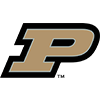Zach Edey
65.1% implied probability

+2000
20 to 1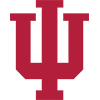Trayce Jackson-Davis
3.2% implied probability

+2500
25 to 1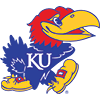Jalen Wilson
2.6% implied probability

+10000
100 to 1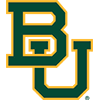Adam Flagler
0.7% implied probability

+10000
100 to 1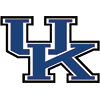Sahvir Wheeler
0.7% implied probability

No changes have been recorded yet.
+10000
100 to 1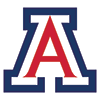Azuolas Tubelis
0.7% implied probability

+10000
100 to 1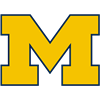Hunter Dickinson
0.7% implied probability

+10000
100 to 1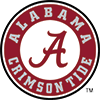Charles Bediako
0.7% implied probability

No changes have been recorded yet.
+10000
100 to 1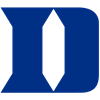Jeremy Roach
0.7% implied probability

+10000
100 to 1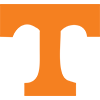Josiah-Jordan James
0.7% implied probability

+10000
100 to 1Dereck Lively
0.7% implied probability

+10000
100 to 1Dariq Whitehead
0.7% implied probability

+10000
100 to 1Kevin McCullar
0.7% implied probability

+10000
100 to 1Cason Wallace
0.7% implied probability

+10000
100 to 1Brandon Miller
0.7% implied probability

+10000
100 to 1Keyonte George
0.7% implied probability

+10000
100 to 1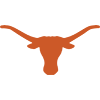Marcus Carr
0.7% implied probability

+10000
100 to 1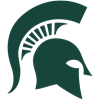Joey Hauser
0.7% implied probability

No changes have been recorded yet.
+10000
100 to 1Adama Sanogo
0.7% implied probability

+15000
150 to 1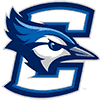Ryan Kalkbrenner
0.4% implied probability

+15000
150 to 1Julian Strawther
0.4% implied probability

+15000
150 to 1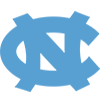R.J. Davis
0.4% implied probability

+15000
150 to 1Jordan Walker
0.4% implied probability

+15000
150 to 1Posh Alexander
0.4% implied probability

+15000
150 to 1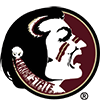Matt Cleveland
0.4% implied probability

+15000
150 to 1Tyrese Hunter
0.4% implied probability

+15000
150 to 1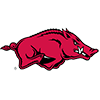Nick Smith
0.4% implied probability

+15000
150 to 1Gradey Dick
0.4% implied probability

+15000
150 to 1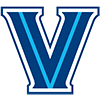Cam Whitmore
0.4% implied probability

+15000
150 to 1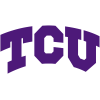Mike Miles
0.4% implied probability

+15000
150 to 1Caleb Love
0.4% implied probability

+15000
150 to 1Santiago Vescovi
0.4% implied probability

No changes have been recorded yet.
+15000
150 to 1Justin Moore
0.4% implied probability

+15000
150 to 1Baylor Scheierman
0.4% implied probability

+15000
150 to 1Jayden Gardner
0.4% implied probability

+15000
150 to 1Timmy Allen
0.4% implied probability

No changes have been recorded yet.
+15000
150 to 1Drew Timme
0.4% implied probability

+15000
150 to 1Oscar Tshiebwe
0.4% implied probability

+15000
150 to 1Anton Watson
0.4% implied probability

+15000
150 to 1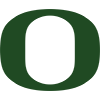Will Richardson
0.4% implied probability

No changes have been recorded yet.
+15000
150 to 1Oumar Ballo
0.4% implied probability

+15000
150 to 1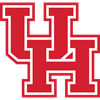Marcus Sasser
0.4% implied probability

+15000
150 to 1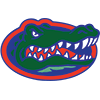Colin Castleton
0.4% implied probability

+20000
200 to 1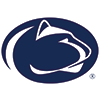Jalen Pickett
0.3% implied probability

+20000
200 to 1Antoine Davis
0.3% implied probability

+20000
200 to 1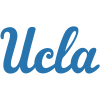Jaylen Clark
0.3% implied probability

+20000
200 to 1Keyontae Johnson
0.3% implied probability

+20000
200 to 1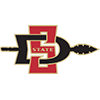Matt Bradley
0.3% implied probability

+20000
200 to 1Tyger Campbell
0.3% implied probability

+20000
200 to 1Kyle Filipowski
0.3% implied probability

+20000
200 to 1Kendric Davis
0.3% implied probability

+20000
200 to 1Leaky Black
0.3% implied probability

+20000
200 to 1Arthur Kaluma
0.3% implied probability

+20000
200 to 1Emoni Bates
0.3% implied probability

+20000
200 to 1Malachi Smith
0.3% implied probability

+20000
200 to 1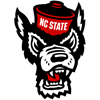Terquavion Smith
0.3% implied probability

+20000
200 to 1DaRon Holmes
0.3% implied probability

+20000
200 to 1Darius McGhee
0.3% implied probability

+20000
200 to 1Armando Bacot
0.3% implied probability

+20000
200 to 1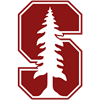Harrison Ingram
0.3% implied probability

+20000
200 to 1Kris Murray
0.3% implied probability

+20000
200 to 1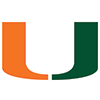Nijel Pack
0.3% implied probability

+20000
200 to 1Isaiah Wong
0.3% implied probability

+20000
200 to 1Jaime Jaquez
0.3% implied probability

+20000
200 to 1Max Abmas
0.3% implied probability

+20000
200 to 1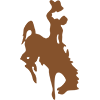Hunter Maldonado
0.3% implied probability

+20000
200 to 1Markquis Nowell
0.3% implied probability